# Floyd Warshall Algorithm

## Introduction

Graphs are one of the most important topics from an interview perspective. Questions related to graphs are frequently asked in interviews of all the major product-based companies. The Floyd Warshall algorithm is for finding the shortest path between all the pairs of vertices in a weighted graph; the algorithm works for both directed and undirected graphs. This algorithm is asked directly in an interview, or the interviewer may give you a real-life situation and will ask you to solve it using graphs.

Before moving on to the algorithm, it is recommended to study Graphs first. This article will describe Floyd Warshall's algorithm with a detailed explanation and code in Java.

## Floyd Warshall Algorithm

Before moving to the jargon of the algorithm, let's understand the problem statement with a real-life example.

Imagine that you(A) and four of your friends (B, C, D, and E) live in different cities/locations. The distance between each of these cities is known. You want to know the optimal path, i.e., the least distance between each of your friend's places. The problem can be solved by representing each of your friend's places and your place by vertices of directed and weighted graphs, as shown below.  The weights between the edges are the distance between vertices of the graph.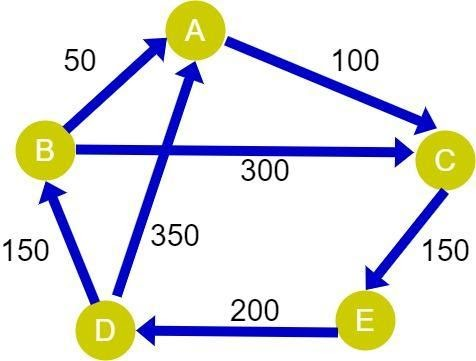Using the Floyd Warshall algorithm, the above problem can be solved very easily. Floyd Warshall will tell you the optimal distance between each pair of friends and will also tell you that the quickest path from friend D to A’s house is via B.

Floyd Warshall algorithm is used to find the shortest path between all the vertices of a directed or undirected weighted graph with no negative cycles.. It is also known as Floyd's algorithm, the Roy-warshall algorithm, the Roy-Floyd algorithm.

This algorithm follows the dynamic programming approach to find the shortest paths. The algorithm doesn't construct the path itself, but it can reconstruct it with a simple modification.

## How does the Floyd Warshall Algorithm Works?

The Floyd algorithm works by initializing the solution matrix the same as the input graph matrix as the first step. The solution matrix, D[][], is then updated by considering all the vertices as an intermediate vertex(say k).

To determine the shortest path between two vertices i and j, there are two possibilities by considering k as the intermediate vertex:

1. If k is not an intermediate vertex, then the value of D[i][j] will remain the same.
2. If k is an intermediate vertex, then the value of D[i][j] will be updated in the following manner:

Consider a directed weighted graph given below to understand the working of the Floyd warshall algorithm.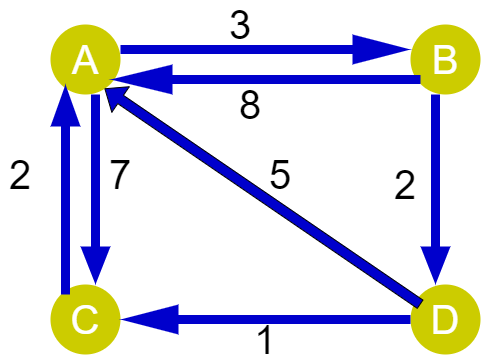Step 1: Create a matrix, D0 of dimensions V*V where V is the number of vertices in the graph. The rows and columns of the matrix are indexed as i and j, respectively, where i and j are the graph's vertices.

Each cell of D0[i][j] is filled with distance from the vertex to the jth vertex. The cell is filled with infinity if there is no path from the vertex to the jth vertex.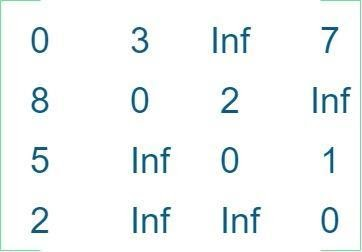Step 2: Now create another matrix D1 using matrix D0. The elements in the first row and the first column are left as it is. The remaining cells are filled in the following manner by considering the 1 as the intermediate vertex or k = 1. Calculate the distance between the source vertex and the destination vertex by considering 1 as the intermediate vertex.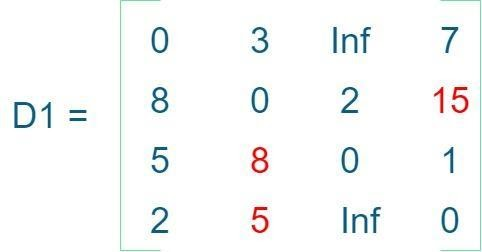Step 3: Similarly, D2 is created using D1. The elements in the second column and the second row are left as it is. The remaining elements are filled by considering the value of k equals 2.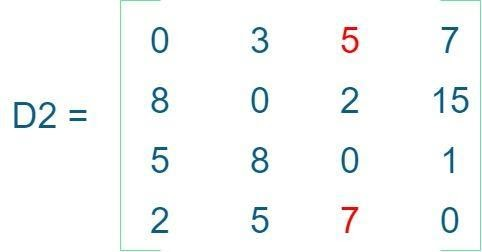Step 4: Create D3 by considering the value of k = 3.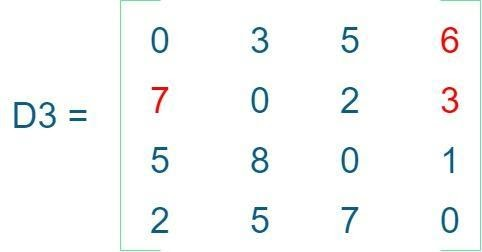Step 5: D4 will give the shortest path between each pair of vertices.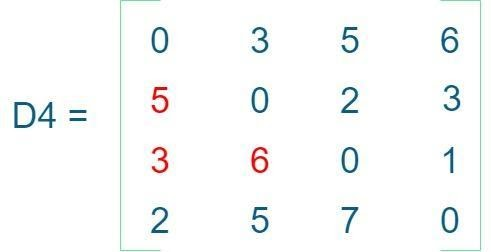## Implementation

The program will make use of the Adjacency Matrix representation of the above graph. The below program shows the implementation of the Floyd Warshall Algorithm in Java.

public class FloydWarshall {

// Using a very large value for the infinity. Note that

// Integer.MAX_VALUE can also be used for this purpose.

final static int INF = 99999;

// Time complexity: O(V^2) for initialization of D[][] and O(V^3) for finding

// the shortest distance

static void floydWarshall(int[][] graph, int vertexCount) {

// The output matrix will be of the same dimensions as

// the original matrix

int[][] D = new int[vertexCount][vertexCount];

// Initializing the output matrix with the same values as

// the input graph

for (int i = 0; i < vertexCount; i++) {

for (int j = 0; j < vertexCount; j++) {

D[i][j] = graph[i][j];

}

}

// Main logic for floyd warshall algorithm. That is, if by

// taking k as the intermediate vertex in between the source,           //i, and destination, j, the

// path length reduces, then D[i][j] will be equal to sum of D[i][k] and

// D[k][j]. Three nested loops are used for this purpose, this makes the

// overall time complexity O(V^3)

for (int k = 0; k < vertexCount; k++) {

for (int i = 0; i < vertexCount; i++) {

for (int j = 0; j < vertexCount; j++) {

if (D[i][k] + D[k][j] < D[i][j]) {

D[i][j] = D[i][k] + D[k][j];

}

}

}

}

// Calling the utility function printMatrix such that the output matrix is

// printed

printMatrix(D);

}

// A utility function to print a matrix

// Time complexity: O(V^2)

static void printMatrix(int[][] matrix) {

for (int i = 0; i < matrix.length; i++) {

for (int j = 0; j < matrix.length; j++) {

System.out.print(matrix[i][j] + " ");

}

System.out.println();

}

}

public static void main(String[] args) {

// Adjacency matrix of the graph

int graph[][] = { { 0, 3, INF, 7 }, { 8, 0, 2, INF }, { 5, INF, 0, INF }, { 2, INF, INF, 0 } };

System.out.println("Printing the original graph");

printMatrix(graph);

System.out.println("\nPrinting the graph after applying Floyd's warshall algorithm");

floydWarshall(graph, graph.length);

}

}

The output of the above program is:

The time complexity of the above program is O(V^3), where V is the count of vertices. The cubic time growth can also be visualized in the following graph.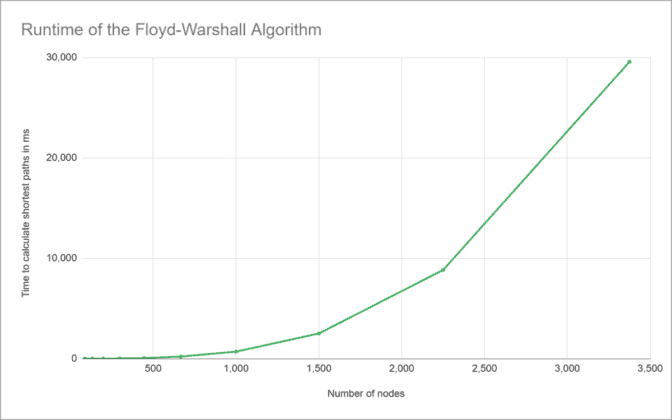(Source: happycoders.eu)

The space complexity of the algorithm is O(V^2).

Note that the above program only prints the shortest distance between edges. However, it can be modified to print the shortest paths by storing the predecessor information in a separate 2D Matrix.

## Applications of Floyd Warshall Algorithm

There are many applications of the Floyd Warshall algorithm. Some of them are given below:

1. It helps in testing whether the graph is bipartite or not.
2. It helps in finding the shortest path in a directed graph.
3. The algorithm helps to find the regular expressions that are accepted by finite automata.
4. It helps in finding the transitive closure of a directed graph.

## Floyd Warshall vs. Dijkstra vs. Bellman-Ford Algorithm

The Dijkstra algorithm is an example of a single-source shortest path algorithm, i.e., it finds the shortest path from a single source vertex to all other vertices. Floyd Warshall, on the other hand, computes the shortest path between all the pairs of vertices. Bellman ford computes the shortest path from a single vertex to all other vertices, and it is capable of solving graphs in which some of the edge weights are negative numbers. The below graph compares the runtime of Floyd Warshall vs. Bellman-Ford vs. Dijkstra Algorithm.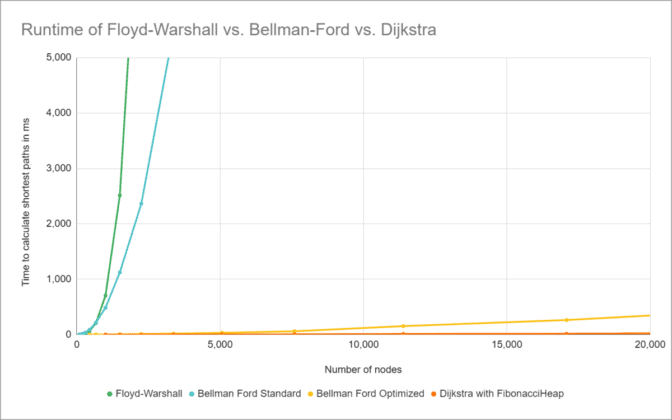(Source: happycoders.eu)

Q1) What is Floyd’s Algorithm used for?

Ans 1) The algorithm is used to find all pair shortest path problems from a given directed or undirected weighted graph. A matrix will be generated that represents the minimum distance from any node to all other nodes in the graph.

Q2) Can Floyd’s warshall algorithm work for an undirected graph?

Ans 2) Every undirected graph can be represented as a directed graph by replacing every edge (i,j) with 2 edges (i,j);(j, i). And if we are running Floyd–Warshall algorithm on such a directed graph - it would work correctly, as always. The algorithm works correctly for both directed and undirected graphs.

Q3) What is the time complexity of Floyd’s algorithm?

Ans 3) Floyd's algorithm is a dynamic programming algorithm used for solving the all-pair shortest path problem. The time complexity of this algorithm is O(V^3), where V is the number of vertices in the graph.

## Key Takeaways

Graphs are an important part of coding interviews. Questions related to graphs are frequently asked in technical interviews of product-based companies. Once you are done with this, it is time to practice more and more questions related to Graphs on Codestudio.  Practice makes perfect !!

You may also check out our interview preparation guided path for preparing for your next interview. Do check out Guided Paths on Codestudio for A complete curated preparation guide for coding interviews in tech and product-based companies. You can choose your tracks and start preparing for your next coding interviews.